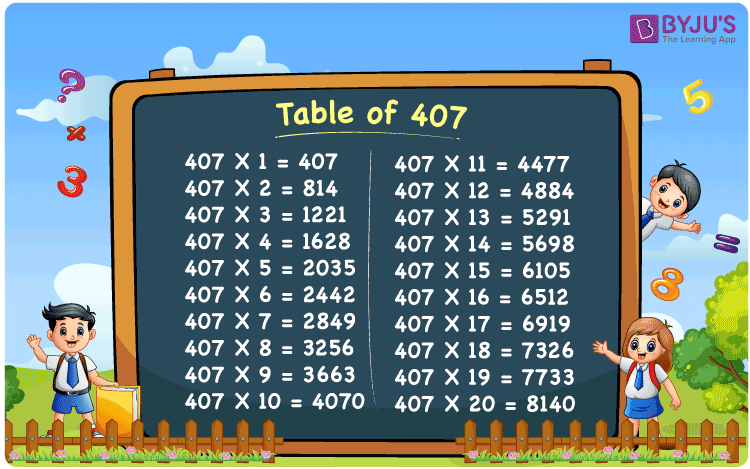Checkout JEE MAINS 2022 Question Paper Analysis : Checkout JEE MAINS 2022 Question Paper Analysis :

# Table of 407

The Table of 407 is provided in the form of a chart which can be accessed by the students absolutely free of cost. Students who are not able to understand the concept behind the multiplication tables can make use of the PDF given at BYJU’S. The topic of repetitive addition, which lies behind the multiplication operation is provided in the form of a table. The multiplication table of 407 PDF can be availed by the students to get their queries clarified without any assistance.

## Table of 407 Chart## What is the 407 Times Table?

The multiplication table of 407 can be used as a reference source by the students while solving the questions of varying difficulty levels. The multiplication and addition operation is explained here in simple language so that students can ace the exams without fear. By having a good idea of these concepts, students will be able to grasp the concepts easily in their higher education.

 407 × 1 = 407 407 407 × 2 = 814 407 + 407 = 814 407 × 3 = 1221 407 + 407 + 407 = 1221 407 × 4 = 1628 407 + 407 + 407 + 407 = 1628 407 × 5 = 2035 407 + 407 + 407 + 407 + 407 = 2035 407 × 6 = 2442 407 + 407 + 407 + 407 + 407 + 407 = 2442 407 × 7 = 2849 407 + 407 + 407 + 407 + 407 + 407 + 407 = 2849 407 × 8 = 3256 407 + 407 + 407 + 407 + 407 + 407 + 407 + 407 = 3256 407 × 9 = 3663 407 + 407 + 407 + 407 + 407 + 407 + 407 + 407 + 407 = 3663 407 × 10 = 4070 407 + 407 + 407 + 407 + 407 + 407 + 407 + 407 + 407 + 407 = 4070

## Multiplication Table of 407

The Table of 407 has to be learnt thoroughly by the students to quickly answer the problems based on multiplication. In the table below, the number 407 is multiplied 20 times which can be referred to by the students to check if their answers are correct. Various competitive exams consider Maths as one of the important subjects, based on which the score will depend on.

 407 × 1 = 407 407 × 2 = 814 407 × 3 = 1221 407 × 4 = 1628 407 × 5 = 2035 407 × 6 = 2442 407 × 7 = 2849 407 × 8 = 3256 407 × 9 = 3663 407 × 10 = 4070 407 × 11 = 4477 407 × 12 = 4884 407 × 13 = 5291 407 × 14 = 5698 407 × 15 = 6105 407 × 16 = 6512 407 × 17 = 6919 407 × 18 = 7326 407 × 19 = 7733 407 × 20 = 8140

## Solved Example on the Table of 407

Q.1: A faculty corrected 19 answer sheets in a day. How many answer sheets will be corrected in 407 days?

Solution: Given,

Number of answer sheets corrected in a day = 19

In 407 days number of answer sheets that will be corrected = 407 x 19

= 7733

## Frequently Asked Questions on the Table of 407

### Find how many times 407 is 8140.

In the table of 407, 407 x 20 = 8140. Therefore, 20 times 407 is 8140.

### Evaluate using 407 times table: 407 multiplied by 12.

From the table of 407, 407 multiplied by 12 is 407 x 12 = 4884.

### Calculate 407 times 10 plus 6 minus 12.

Using the table of 407, 407 times 10 plus 6 minus 12 can be written as 407 x (10 + 6 – 12) = 407 x 4 = 1628